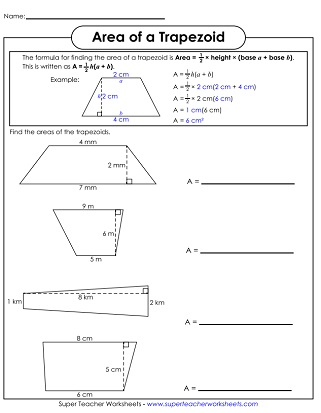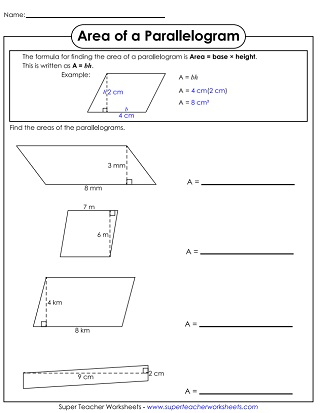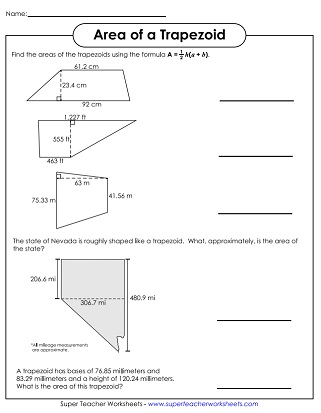# Area of Trapezoids and Parallelograms

This page has a collection of printable area worksheets with parallelograms and trapezoids.## Level: Basic

Use the formula to calculate the areas of the trapezoids shown. This basic version contains small, whole number measurements.
To find the area of each parallelogram, simply multiply the base time the height. This version includes single-digit numbers for the lengths of the sides.
This file contains 30 task cards with parallelograms. Use them for whole group instruction (with document camera), for learning centers, for peer study groups, or for independent practice.
Download a deck of 30 "area of a trapezoid" cards. Use them for peer study groups, classroom scavenger hunts, or small group instruction.

## Level: Intermediate

Use the formula to calculate the area of the three trapezoids shown. In the intermediate-level worksheets, the measurements are all double-digit whole numbers.
Multiply base times height to find the areas of the four parallelograms on this page. Because this is an intermediate-level worksheet, all measurements will be whole numbers with 2 digits.

On this advanced level worksheet, students will find the area of 5 different parallelograms using 3- and 4-digit numbers and decimals.
Find the areas of five different trapezoids. This advanced-level worksheet includes 3-digit numbers and decimals.
More Area Worksheets

Find the areas of all types of shapes, including circles, rectangles, and triangles. There's a section for surface area worksheets too.

Geometry Worksheets

Link to printables on symmetry, tessellations, translation/rotation/reflection, polygons, plotting points, and more.

## Worksheet ImagesMy Account
Site Information